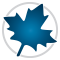Linear Algebra - Maple Features - Maplesoft# Maple

Linear AlgebraMaple has efficient and comprehensive linear algebra support for both small- and large-scale problems. Its linear algebra capabilities include:

• Over 100 commands for constructing, solving, programming, and querying topics in linear algebra.
• Standard 2-D notation to enter matrices and vectors and display results.
• Many operations available through context-sensitive menus and task templates, as well as commands.
• Symbolic and numeric computations, including support for matrices containing both numbers and variables.
• Eigenvalues and eigenvectors, both classical and generalized.
• Sophisticated algorithms for linear algebra over finite fields.
• Many matrix factorizations and system solvers.
• State-of-the-art numerical methods for dense and sparse systems with a high degree of user control.
• Support for both hardware float and arbitrary precision software float data.
• Numeric routines from CLAPACK and optimized vendor BLAS (ATLAS and MKL) libraries. These routines have been integrated into Maple and are called automatically when appropriate.

Maple also contains numerous tools for teaching and learning linear algebra concepts:

• Interactive tutors let you single step through Gaussian elimination; Gauss- Jordan elimination; and the computation of the inverse, eigenvalues, and eigenvectors of a matrix.
• Visualization routines display the cross product of two vectors, a system of 2-D or 3-D linear equations, the projection of a vector onto a subspace, and more.
• Over 25 task templates assist in common linear algebra operations, including taking cross and dot products, calculating bases, and solving systems of linear equations.
Prêt(e) pour l'étape suivante ?

*Ce programme d'évaluation n'est proposé actuellement ni aux étudiants ni aux particuliers.

Achetez: